# asymptotics

Determine Markov chain asymptotics

## Syntax

``xFix = asymptotics(mc)``
``[xFix,tMix] = asymptotics(mc)``

## Description

example

``xFix = asymptotics(mc)` returns the stationary distribution `xFix` of the discrete-time Markov chain `mc`.`

example

``[xFix,tMix] = asymptotics(mc)` additionally returns an estimate of the mixing time `tMix`.`

## Examples

collapse all

Consider this theoretical, right-stochastic transition matrix of a stochastic process.

`$P=\left[\begin{array}{ccccccc}0& 0& 1/2& 1/4& 1/4& 0& 0\\ 0& 0& 1/3& 0& 2/3& 0& 0\\ 0& 0& 0& 0& 0& 1/3& 2/3\\ 0& 0& 0& 0& 0& 1/2& 1/2\\ 0& 0& 0& 0& 0& 3/4& 1/4\\ 1/2& 1/2& 0& 0& 0& 0& 0\\ 1/4& 3/4& 0& 0& 0& 0& 0\end{array}\right].$`

Create the Markov chain that is characterized by the transition matrix P.

```P = [ 0 0 1/2 1/4 1/4 0 0 ; 0 0 1/3 0 2/3 0 0 ; 0 0 0 0 0 1/3 2/3; 0 0 0 0 0 1/2 1/2; 0 0 0 0 0 3/4 1/4; 1/2 1/2 0 0 0 0 0 ; 1/4 3/4 0 0 0 0 0 ]; mc = dtmc(P);```

Plot a directed graph of the Markov chain. Indicate the probability of transition by using edge colors.

```figure; graphplot(mc,'ColorEdges',true);```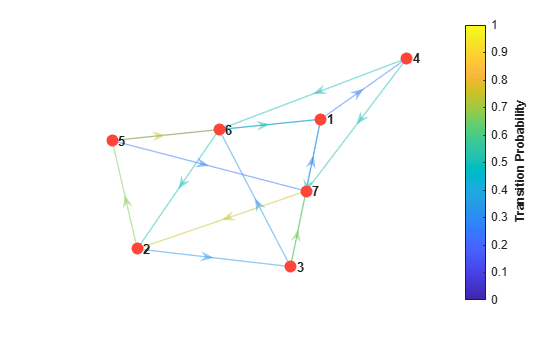Determine the stationary distribution of the Markov chain.

`xFix = asymptotics(mc)`
```xFix = 1×7 0.1300 0.2034 0.1328 0.0325 0.1681 0.1866 0.1468 ```

Because `xFix` is a row vector, it is the unique stationary distribution of `mc`.

Create a five-state transition matrix of empirical counts by generating a block diagonal matrix composed of discrete uniform draws.

```m = 100; % Maximal count rng(1); % For reproducibility P = blkdiag(randi(100,2) + 1,randi(100,3) + 1)```
```P = 5×5 43 2 0 0 0 74 32 0 0 0 0 0 16 36 43 0 0 11 41 70 0 0 20 55 22 ```

Create and plot a digraph of the Markov chain that is characterized by the transition matrix P.

```mc = dtmc(P); figure; graphplot(mc)```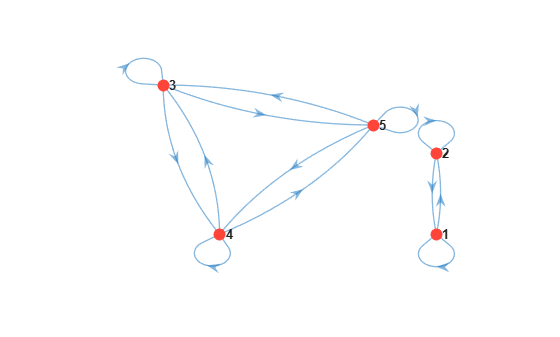Determine the stationary distribution and mixing time of the Markov chain.

`[xFix,tMix] = asymptotics(mc)`
```xFix = 2×5 0.9401 0.0599 0 0 0 0 0 0.1497 0.4378 0.4125 ```
```tMix = 0.8558 ```

Rows of `xFix` correspond to the stationary distributions of the two independent recurrent classes of `mc`.

Create separate Markov chains representing the recurrent subchains of `mc`.

```mc1 = subchain(mc,1); mc2 = subchain(mc,3);```

`mc1` and `mc2` are `dtmc` objects. `mc1` is the recurrent class containing state `1`, and `mc2` is the recurrent class containing state `3`.

Compare the mixing times of the subchains.

`[x1,t1] = asymptotics(mc1)`
```x1 = 1×2 0.9401 0.0599 ```
```t1 = 0.7369 ```
`[x2,t2] = asymptotics(mc2)`
```x2 = 1×3 0.1497 0.4378 0.4125 ```
```t2 = 0.8558 ```

`mc1` approaches its stationary distribution more quickly than `mc2`.

Create a "dumbbell" Markov chain containing 10 states in each "weight" and three states in the "bar."

• Specify random transition probabilities between states within each weight.

• If the Markov chain reaches the state in a weight that is closest to the bar, then specify a high probability of transitioning to the bar.

• Specify uniform transitions between states in the bar.

```rng(1); % For reproducibility w = 10; % Dumbbell weights DBar = [0 1 0; 1 0 1; 0 1 0]; % Dumbbell bar DB = blkdiag(rand(w),DBar,rand(w)); % Transition matrix % Connect dumbbell weights and bar DB(w,w+1) = 1; DB(w+1,w) = 1; DB(w+3,w+4) = 1; DB(w+4,w+3) = 1; mc = dtmc(DB);```

Visualize the transition matrix using a heatmap.

```figure; imagesc(mc.P); colormap(jet); axis square; colorbar;```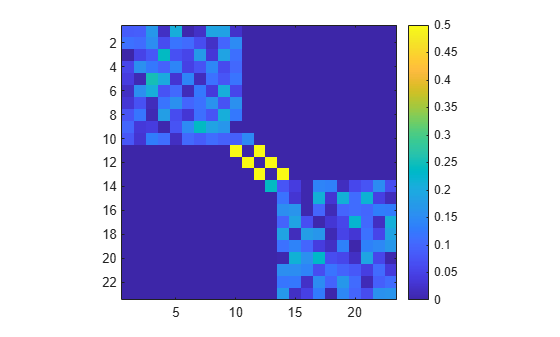Plot a directed graph of the Markov chain. Suppress node labels.

```figure; h = graphplot(mc); h.NodeLabel = {};```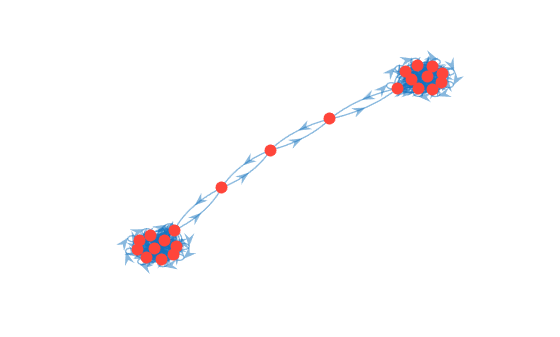Plot the eigenvalues of the dumbbell chain.

```figure; eigplot(mc);```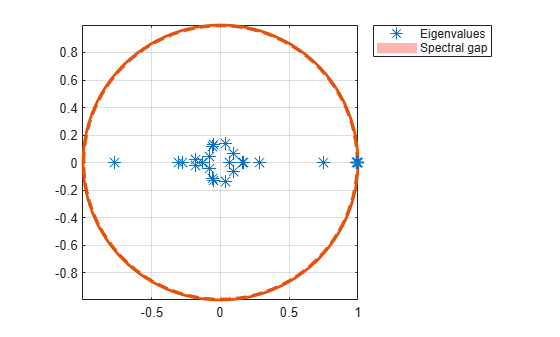The thin, red disc in the plot shows the spectral gap (the difference between the two largest eigenvalue moduli). The spectral gap determines the mixing time of the Markov chain. Large gaps indicate faster mixing, whereas thin gaps indicate slower mixing. In this case, the spectral gap is thin, indicating a long mixing time.

Estimate the mixing time of the dumbbell chain and determine whether the chain is ergodic.

`[~,tMix] = asymptotics(mc)`
```tMix = 85.3258 ```
`tf = isergodic(mc)`
```tf = logical 1 ```

On average, the time it takes for the total variation distance between any initial distribution and the stationary distribution to decay by a factor of ${e}^{1}$ is about 85 steps.

## Input Arguments

collapse all

Discrete-time Markov chain with `NumStates` states and transition matrix `P`, specified as a `dtmc` object. `P` must be fully specified (no `NaN` entries).

## Output Arguments

collapse all

Stationary distribution, with `xFix*P` = `xFix`, returned as a nonnegative numeric matrix with `NumStates` columns. The number of rows of `xFix` is the number of independent recurrent classes in `mc`.

• For unichains, the distribution is unique, and `xFix` is a `1`-by-`NumStates` vector.

• Otherwise, each row of `xFix` represents a distinct stationary distribution in `mc`.

Mixing time, returned as a positive numeric scalar.

If μ, the second largest eigenvalue modulus (SLEM) of `P`, exists and is nonzero, then the estimated mixing time is $-1/\mathrm{log}\left(\mu \right)$.

Note

• If `P` is a nonnegative stochastic matrix, then the Markov chain `mc` it characterizes has a left eigenvector `xFix` with eigenvalue `1`. The Perron-Frobenius Theorem  implies that if `mc` is a unichain (a chain with a single recurrent communicating class), then `xFix` is unique. For reducible chains with multiple recurrent classes, eigenvalue `1` has higher multiplicity, and `xFix` is nonunique. If a chain is periodic, `xFix` is stationary but not limiting because arbitrary initial distributions do not converge to it. `xFix` is both unique and limiting for ergodic chains only. See `classify`.

• For ergodic chains, `tMix` is a characteristic time for any initial distribution to converge to `xFix`. Specifically, it is the time for the total variation distance between an initial distribution and `xFix` to decay by a factor of `e` = `exp(1)`. Mixing times are a measure of the relative connectivity of transition structures in different chains.

 Gallager, R.G. Stochastic Processes: Theory for Applications. Cambridge, UK: Cambridge University Press, 2013.

 Horn, R., and C. R. Johnson. Matrix Analysis. Cambridge, UK: Cambridge University Press, 1985.

 Seneta, E. Non-negative Matrices and Markov Chains. New York, NY: Springer-Verlag, 1981.